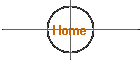### Our Sun, The Nearest Star

 Distance from Earth 93 million miles, 8.5 light minutes Diameter 864,000 miles Mass 330,000 x Earth Diameter 109 x Earth Age 4.5 billon years Fate Red Giant in 5 billion years Rotation at equator 25 Earth days Rotation at poles 35 Earth days Solar sunspot cycle 11 Years Temperature at core 27,000,000 degrees F Temperature at surface 10,000 degrees F Temperature of sunspots 7,300 degrees F

The sun turns 700,000,000 tons of hydrogen into 695,000,000 tons of helium every second by nuclear fusion. The remaining 5 tons is converted into energy. 400,000,000,000,000,000,000,000,000 watts

### Distances to Other Stars

Distances are particularly easy to calculate if we use the parsec as our distance unit. In that case, the distance of a star in parsecs is:

D = 1/p

where D is the distance in pc and p is the parallax angle in seconds  of arc. For example, Sirius has a parallax angle of 0.38 seconds of arc  and thus its distance from the Earth is d = 1/0.38 = 2.6 pc = 8.6 LY.   The nearest star (other than the Sun) is the alpha-Centauri system,  which has a parallax of 0.76 seconds of arc, corresponding to a  distance of 1.315 pc = 4.3 LY. Thus, all stars have parallax angles of  less than one second of arc.

### The Nearest Stars

 `Name` `Dist``(LY)` `Spectral``Type` `R.A.` `Dec.` `Luminosity``(Solar Units)` `Proxima Centauri` `4.2` `M5V` `14 30` `-62 41` `6 x 10-6` `Alpha Centauri A` `4.3` `G2V` `14 33` `-60 50` `1.5` `Alpha Centauri B` `4.3` `K0V` `14 33` `-60 50` `0.5` `Barnard's Star` `6.0` `M4V` ` 17 57` `+04 33` `4 x 10-4` `Wolf 359 (Gliese 406)` `7.8` `M6V` `10 56` `+07 03` `2 x 10-5` `Lalande 21185 (HD 95735)` `8.2` `M2V` `11 04` `+36 02` `5 x 10-3` `Luyten 726-8 A` `8.6` `M5V` `01 38` `-17 58` `6 x 10-5` `Luyten 726-8 B (UV Ceti)` `8.6` `M6V` `01 38` `-17 58` `4 x 10-5` `Sirius A` `8.6` `A1V` `06 45` `-16 43` `24` `Sirius B` `8.6` `WD` `06 45` `-16 43` `3 x 10-3` `Ross 154 (Gliese 729)` `9.6` `M4V` `18 50` `-23 49` `5 x 10-4` `Ross 248 (Gliese 905)` `10.3` `M6V` `23 42` `+44 12` `1 x 10-4` `Epsilon Eridani` `10.7` `K2V` `03 33` `-09 27` `0.3` `Ross 128 (Gliese 447)` `10.8` `M4V` `11 48` `+00 49` `3 x 10-4` `Luyten 789-6 A1` `11.1` `M5V` `22 39` `-15 20` `1 x 10-4` `Luyten 789-6 B` `11.1` `22 39` `-15 20` `Luyten 789-6 C` `11.1` `22 39` `-15 20` `BD +43 44 A (Gliese 15 A)` `11.3` `M1V` `00 18` `+44 61` `6 x 10-3` `BD +43 44 B (Gliese 15 B)` `11.3` `M3V` `00 18` `+44 61` `4 x 10-4` `Epsilon Indi` `11.3` `K5V` `22 03` `-56 47` `0.14` `61 Cygni A` `11.3` `K5V` `21 07` `+38 45` `0.008` `61 Cygni B` `11.3` `K7V` `21 07` `+38 45` `0.004` `BD +59 1915 A (Gliese 725 A)` `11.4` `M3V` `18 43` `+59 37` `0.003` `BD +59 1915 B (Gliese 725 B)` `11.4` `M4V` `18 43` `+59 37` `0.002 `

### How far away is Polaris? Where can I find reliable distances to nearby stars?

I am trying to find out the distance to the pole star. In one reference I found on the web 300 ly was the figure given while a second source gave 690 ly.  My question for you is what is the distance and is there a > generally accepted reference available on line to get such information?